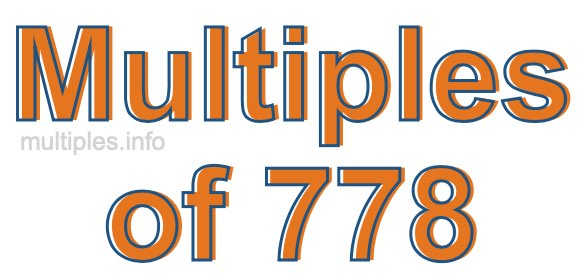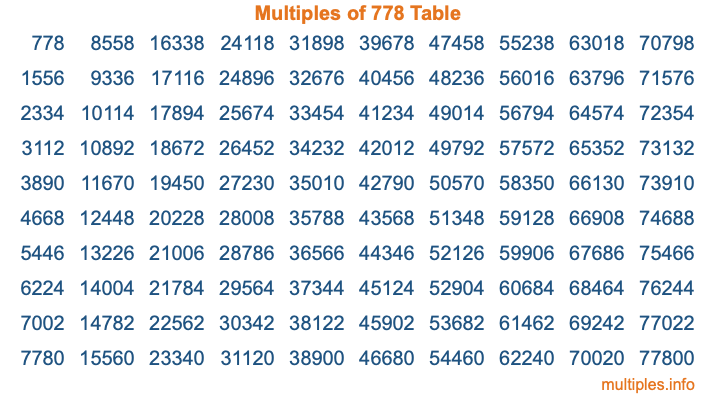Multiples of 778Welcome to the Multiples of 778 page. Here we will first teach you everything you will ever need to know about the multiples of 778, and then give you a study guide summary of everything we taught you to make sure you remember it all. Use this page to look up facts and learn information about the multiples of 778. This page will make you a multiples of seven hundred seventy-eight expert!

Definition of Multiples of 778
Multiples of 778 are all the numbers that when divided by 778 equal an integer. Each of the multiples of 778 are called a multiple. A multiple of 778 is created by multiplying 778 by an integer.

Therefore, to create a list of multiples of 778, you start with 1 multiplied by 778, then 2 multiplied by 778, then 3 multiplied by 778, and so on for as long as you want. Thus, the list of the first five multiples of 778 is 778, 1556, 2334, 3112, and 3890. To see a larger list of multiples of 778, see the printable image of Multiples of 778 further down on this page. We also have a category where you can choose any nth multiple of 778.

Multiples of 778 Checker
The Multiples of 778 Checker below checks to see if any number of your choice is a multiple of 778. In other words, it checks to see if there is any number (integer) that when multiplied by 778 will equal your number. To do that, we divide your number by 778. If the the quotient is an integer, then your number is a multiple of 778.

Is  a multiple of 778?

Least Common Multiple of 778 and ...
A Least Common Multiple (LCM) is the lowest multiple that two or more numbers have in common. This is also called the smallest common multiple or lowest common multiple and is useful to know when you are adding our subtracting fractions. Enter one or more numbers below (778 is already entered) to find the LCM.

Check out our LCM Calculator if you need more details about the Least Common Multiple or if you need the LCM for different numbers for adding and subtraction fractions.

nth Multiple of 778
As we stated above, 778 is the first multiple of 778, 1556 is the second multiple of 778, 2334 is the third multiple of 778, and so on. Enter a number below to find the nth multiple of 778.

th multiple of 778

Multiples of 778 vs Factors of 778
778 is a multiple of 778 and a factor of 778, but that is where the similarities end. All postive multiples of 778 are 778 or greater than 778. All positive factors of 778 are 778 or less than 778.

Below is the beginning list of multiples of 778 and the factors of 778 so you can compare:

Multiples of 778: 778, 1556, 2334, 3112, 3890, etc.

Factors of 778: 1, 2, 389, 778

As you can see, the multiples of 778 are all the numbers that you can divide by 778 to get a whole number. The factors of 778, on the other hand, are all the whole numbers that you can multiply by another whole number to get 778.

It's also interesting to note that if a number (x) is a factor of 778, then 778 will also be a multiple of that number (x).

Multiples of 778 vs Divisors of 778
The divisors of 778 are all the integers that 778 can be divided by evenly. Below is a list of the divisors of 778.

Divisors of 778: 1, 2, 389, 778

The interesting thing to note here is that if you take any multiple of 778 and divide it by a divisor of 778, you will see that the quotient is an integer.

Multiples of 778 Table
Below is an image of the first 100 multiples of 778 in a table. The table is in chronological order, column by column. The first column has the first ten multiples of 778, the second column has the next ten multiples of 778, and so on.The Multiples of 778 Table is also referred to as the 778 Times Table or Times Table of 778. You are welcome to print out our table for your studies.

Negative Multiples of 778
Although not often discussed or needed in math, it is worth mentioning that you can make a list of negative multiples of 778 by multiplying 778 by -1, then by -2, then by -3, and so on, to get the following list of negative multiples of 778:

-778, -1556, -2334, -3112, -3890, etc.

Multiples of 778 Summary
Below is a summary of important Multiples of 778 facts that we have discussed on this page. To retain the knowledge on this page, we recommend that you read through the summary and explain to yourself or a study partner why they hold true.

There are an infinite number of multiples of 778.

A multiple of 778 divided by 778 will equal a whole number.

778 divided by a factor of 778 equals a divisor of 778.

The nth multiple of 778 is n times 778.

The largest factor of 778 is equal to the first positive multiple of 778.

778 is a multiple of every factor of 778.

778 is a multiple of 778.

A multiple of 778 divided by a divisor of 778 equals an integer.

778 divided by a divisor of 778 equals a factor of 778.

Any integer times 778 will equal a multiple of 778.

Multiples of a Number
Here you can get the multiples of another number, all with the same attention to detail as we did for multiples of 778 on this page.

Multiples of
Multiples of 779
Did you find our page about multiples of seven hundred seventy-eight educational? Do you want more knowledge? Check out the multiples of the next number on our list!